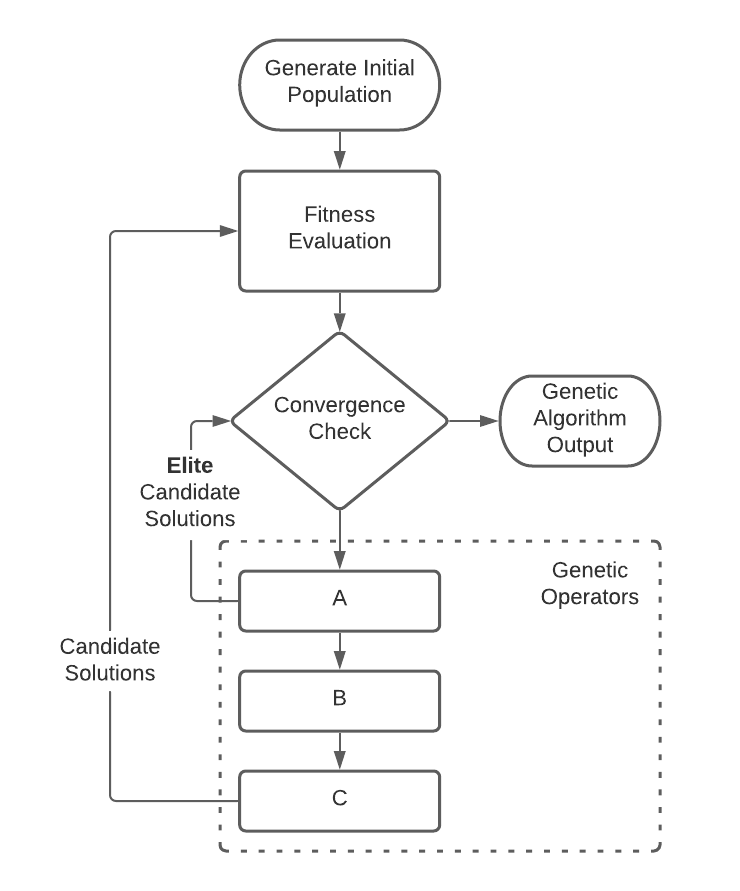Login or Create an Account to view the mark scheme, comment, and add to a testWith respect to the Genetic Algorithm (GA) flow diagram above, select the option below which correctly specifies the genetic operations applied in a typical GA process.
(a).
A = Crossover, B = Mutation, C = Selection
(b).
A = Exploitation, B = Selection, C = Mutation
(c).
A = Selection, B = Crossover, C = Mutation
(d).
A = Crossover, B = Mutation, C = Exploration

HomeThe Right Test - Fast